### RIGHT Function Examples – Excel, VBA, & Google Sheets

This tutorial demonstrates how to use the Excel RIGHT Function in Excel to return text from the end of a cell.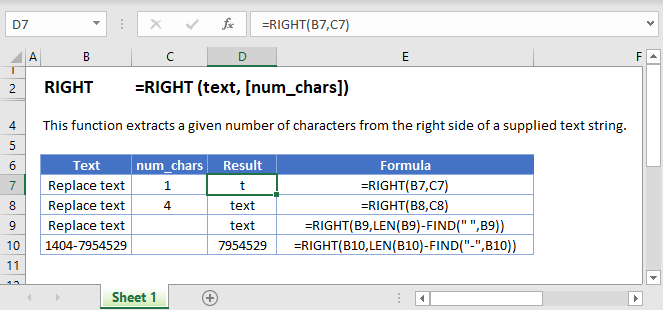## RIGHT Function Overview

The RIGHT Function Returns a specified number of characters from the end of a cell.

To use the RIGHT Excel Worksheet Function, select a cell and type: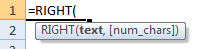(Notice how the formula inputs appear)

### RIGHT function Syntax and inputs:

`=RIGHT(text,num_chars)`

text – A string of text.

num_chars – The number of characters from the end of the cell to return.

AutoMacro - VBA Code Generator

## How to use the RIGHT Function in Excel:

The RIGHT function extracts a specific number of characters you specify from the RIGHT (end of the string).

`=RIGHT(B3,C3)`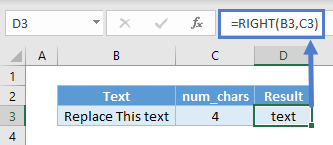The above extracts 4 characters from the RIGHT or the end of the string.

`=RIGHT(B3)`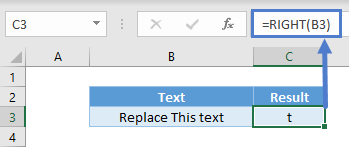The second argument is optional and the default value of it is 1. Hence, it extracts 1 character from the RIGHT and gives you “t” if you omit it.

## RIGHT with FIND/SEARCH and LEN

In many scenarios, the number of characters we want to extract from the RIGHT varies just like last names.

`=RIGHT(B3,LEN(B3)-FIND(" ",B3))`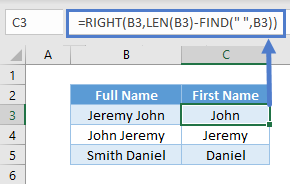In this case here, we can use FIND to check which character position the space starts. It also represents how many characters the first name and space have in total.

LEN helps us obtain the total number of characters in the cell. Using LEN minus first name and space total characters would give us the number of characters to extract from the right and give us the last name.

You can also use SEARCH. The difference between FIND and SEARCH is that the former is case-sensitive. Finding a symbol such as space wouldn’t matter.

`=RIGHT(B3,SEARCH(" ",B3)-1)`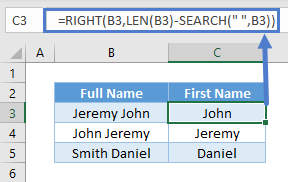## RIGHT with LEN

There could be instances you know how many characters you don’t want, but not how many you want. In the eg below, there are always 2 alphabets in front. But the numbers differ.

`=RIGHT(B3,LEN(B3)-2)`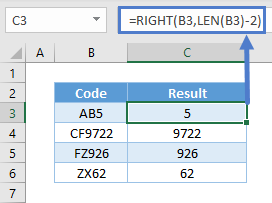Since we know we don’t want the 2 alphabets in front, simply use total length minus 2 characters to obtain the numbers behind.

## RIGHT with Number/Dates

Do note that RIGHT is a text function. Upon using RIGHT, the result is a text. For instance, you won’t be able to sum up these numbers in cell E3 after using RIGHT.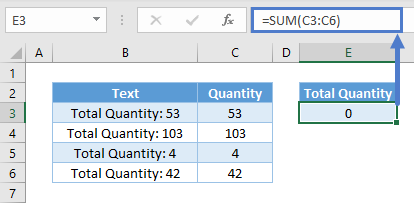The same thing happens for dates as they are recognized as serial numbers and not text. You may not need to sum dates, but it doesn’t work well in filters and PivotTables.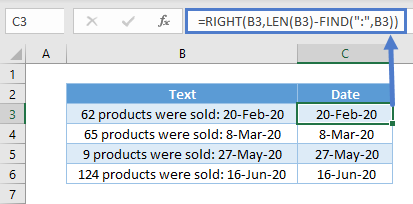To overcome the issues above, you can use VALUE to convert from text to values.

`=VALUE(RIGHT(B3,LEN(B3)-LEN("Total Quantity: ")))`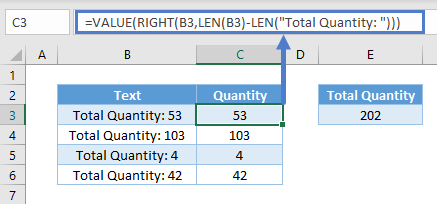`=VALUE(RIGHT(B3,LEN(B3)-FIND(":",B3)))`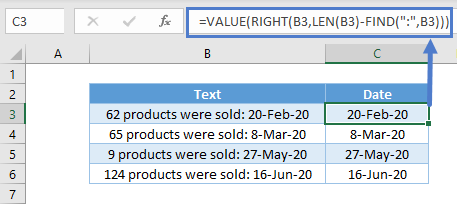# Useful Right  function examples

You can combine RIGHT with some of the Excel functions in some useful ways.

## Extract number from the Right of a string

When you working with excel you need to exact the number from given detail. Sometime you need to exact number from Left and sometime you need to extract number from Right. So RIGHT function will help you to do this task by combining with few other functions.

### Extract number from the Right

We could combine LEN , MIN, FIND with RIGHT as follows:

`=RIGHT(A2,LEN(A2)-MIN(FIND({0,1,2,3,4,5,6,7,8,9},A2&"0123456789"))+1)`

Which would give us the following results: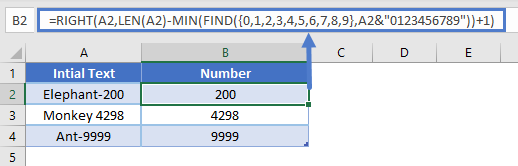### Apply leading ZEROS to number by using Right function

Add leading ZEROS is very useful when you working with excel. In this example we will explain how you can do this by combine RIGHT with CONCATENATE function.

In above example we explain add leading zeros only till 7 digits, but based on the requirement you can change number 7  and no of Zeros to get required out put. you can refer below example to get leading zero up to 11 digits.

`=RIGHT(CONCATENATE("0000000000",A2), 11)`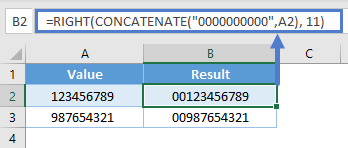### More Examples for Add leading ZEROS to number.

Below table help you give more ways for add leading zeros to set number.### Extract last word from String

We could combine LEFT, SUM, SUBSTITUTE with LEN as follows:

Which would give us the following results: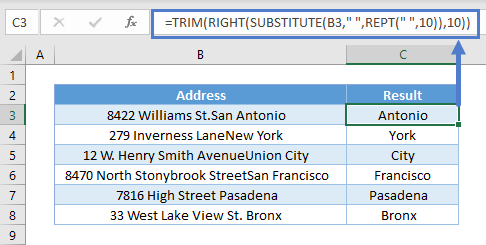This function set for less than 10 word, so if you need to find out last word for long string you can change this number 10.

## RIGHT in Google Sheets

The RIGHT Function works exactly the same in Google Sheets as in Excel: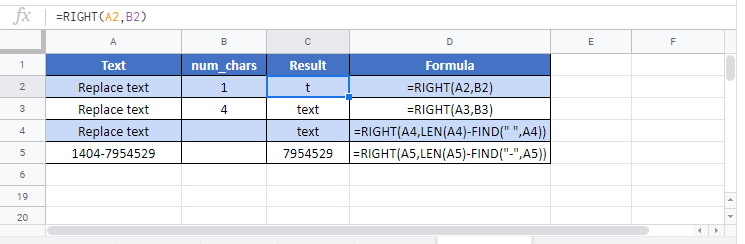## Additional Notes

If num_chars is greater than the length of the text, the RIGHT Function returns all text.

## RIGHT Examples in VBA

You can also use the RIGHT function in VBA. Type:
`application.worksheetfunction.right(text,num_chars)`
For the function arguments (text, etc.), you can either enter them directly into the function, or define variables to use instead.

Return to the List of all Functions in Excel

## Excel Practice Worksheet

Practice Excel functions and formulas with our 100% free practice worksheets!

• Automatically Graded Exercises
• Learn Excel, Inside Excel!